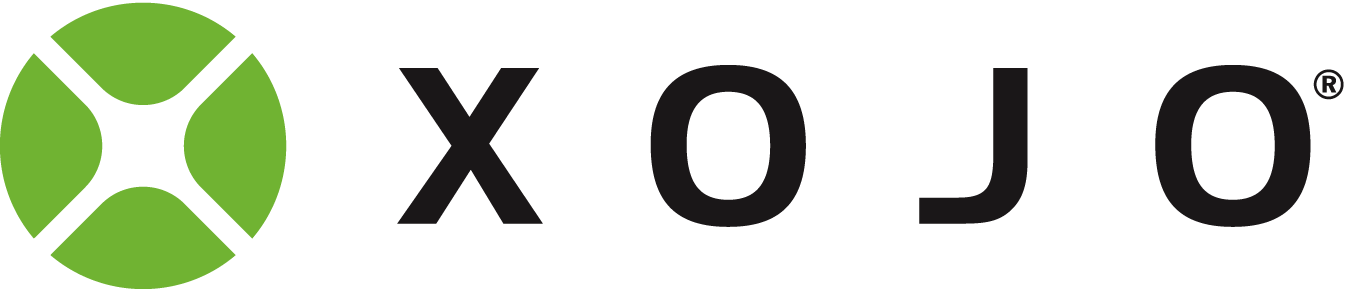# Very odd math mismatch when using IF...THEN

It’s not negative. It’s 10000000000000000000 the first time and multiplied by 2 every other time.
And why does it work correct when multiplying with even values and not with uneven?

There is definitely something wrong with Xojo math or the IF…THEN procedure.

EDIT: changing to UINT64 does also not resolve the issue.

``````Dim timeNano1 as int64
timeNano1= 10000000000000000000
for a as integer = 1 to 10
if (timeNano1*a) > CType(0, Int64) then
msgbox "Is bigger than zero @ a="+str(a)
end
next a
``````

Try the above
This is compile comparison error cause you have Int64, Integer and comparing to Integer

i get output:

``````       Is bigger than zero @ a=2
Is bigger than zero @ a=4
Is bigger than zero @ a=6
Is bigger than zero @ a=8
Is bigger than zero @ a=10``````

If I put a breakpoint after the second line, the debugger shows -8446744073709551616.

The largest number you can store in an Int64 is 9223372036854775807.

10000000000000000000 is larger than 9223372036854775807.

Try with UINT64 … that can hold higher values but the issue remains.

@ DerkJ
Bummer … that does fix the issue. But why do I need use CType(0, Int64) and why does plain ‘0’ does not work? This smells like a Xojo problem to me.

I did search the forum I am apparently not alone with this problem.
This thread tells me it IS a Xojo problem - likely a compiler problem.

1 Like

It does NOT fix it.

Since @keving is right, it’s coming out negative.

``````       -8446744073709551616 - NOT bigger than zero @ a=1
1553255926290448384 - Is bigger than zero @ a=2
-6893488147419103232 - NOT bigger than zero @ a=3
3106511852580896768 - Is bigger than zero @ a=4
-5340232221128654848 - NOT bigger than zero @ a=5
4659767778871345152 - Is bigger than zero @ a=6
-3786976294838206464 - NOT bigger than zero @ a=7
6213023705161793536 - Is bigger than zero @ a=8
-2233720368547758080 - NOT bigger than zero @ a=9``````

It seems to be a compiler issue.

use CType to work around it if you can.

If i use only Uint64 i get this output:
10000000000000000000 - Is bigger than zero @ a=1
1553255926290448384 - Is bigger than zero @ a=2
11553255926290448384 - Is bigger than zero @ a=3
3106511852580896768 - Is bigger than zero @ a=4
13106511852580896768 - Is bigger than zero @ a=5
4659767778871345152 - Is bigger than zero @ a=6
14659767778871345152 - Is bigger than zero @ a=7
6213023705161793536 - Is bigger than zero @ a=8
16213023705161793536 - Is bigger than zero @ a=9
7766279631452241920 - Is bigger than zero @ a=10

Which seems very strange since aVal * LoopNum should do it * 1 or * 2 etc…

EDIT: it’s not strange since it’s overflowing…10000000000000000000 - Is bigger than zero @ a=1
18446744073709551615 - is the UInt64 max

since 10000000000000000000 * 2 = 20000000000000000000
and that’s higher than the max… hence the overflow.

I do see some kind of bug when timeNano1 is UInt64.

I suggest you log it in Feedback.

That’s a known compiler issue. Since the Xojo compiler is not being smart here.

``````TimeNano1 = Int64
a = Integer
> 0 = Integer
``````

Compiler is not changing the >0 to Int64… so you get trouble (since the sign is not accounted for)

Maybe the correct question is why it reverts to negative when it overflows. Anyhow using CType is a workaround. It fixes the problem in my app. Thx

This crops up when people use timers in apps that run for days and weeks.
They want to know how long the app has been open, or time between two points.
A single Integer (no matter how large) is not good for this because sooner or (much) later it overflows and you end up with an apparent ‘used for - 3000 years’

If you need such a timer, create a method or class that has a value for Days_Elapsed as UINT64 or even a double.

Have your timer by all means, but once it reaches a value that means 24 hours, go increment the days counter, and reset your ticks/seconds variable to 0

Doing it that way means you will not overflow before the Sun explodes.

Well, that’s something Xojo Inc should fix. It is prone for difficult bugs to track. I lost several days why the maths did not work. I though I was losing my mind.2 Likes
``````Dim timeNano1 As UInt64 = 10000000000000000000
Var maxUint64 As UInt64 = -1 // This gives the max value since it will back-overflow

Var aVal, calc As UInt64

For a As UInt64 = 1 To 10

aVal = A
calc = (timeNano1 * aVal)

If calc > CType(0, UInt64) Then
System.DebugLog calc.ToString + " - Is bigger than zero @ a=" + a.ToString
Else
System.DebugLog calc.ToString + " - NOT bigger than zero @ a=" + a.ToString
End

// max Uit64:
System.DebugLog maxUint64.ToString +" is the UInt64 max"

Next a
``````

Even this is (UInt64) not working, it seems it’s still handled as Int64 probably in .ToString ?
This smells like a bug, try the output it’s the same as if all was an int64 while it’s not.

Probably @Thom_McGrath knows what’s happening here?